Articulo

•Citado por SciELO
•Accesos

•Similares en SciELO

versión On-line ISSN 2074-4706

rlde  n.5 La Paz oct. 2005

TRABAJOS DE INVESTIGACIÓN

Measuring Willingness-to-Pay in Discrete Choice Models with Semi-Parametric Techniques

Pablo Marcelo García*

Abstract

It is usual to estimate willingness-to-pay in discrete choice models through Logit models -or their expanded versions. Nevertheless, these models have very restrictive distributional assumptions. This paper is intended to examine the above-mentioned issue and to propose an alternative estimation using non-parametric techniques (through Simple Index Models). Furthermore, this paper introduces an empirical application of willingness-to-pay for improved subway travel times in the City of Buenos Aires.

Resumen**

Es usual estimar la disposición a pagar por mejoras en modelos de elección discreta a través de modelos logit -o extensiones del mismo. Sin embargo, este tipo de modelos posee supuestos distribucionales muy restrictivos. El presente trabajo explora este tipo de problemas y propone una estimación alternativa utilizando técnicas no paramétricas (mediante Simple Index Models). Adicionalmente, se realiza una aplicación empírica a la disposición a pagar por mejoras en los tiempos de viaje en subterráneo en la ciudad de Buenos Aires.

1. Introduction

It is usual to estimate willingness-to-pay in discrete choice models through Logit models -or their expanded versions1. Nevertheless, this type of models has distributional assumptions which, if not fulfilled, can lead to considerable estimation bias. This paper is intended to examine the subject above proposing an alternative estimation through non-parametric techniques rather than through Logit models.

In order to achieve that goal, a brief description regarding discrete choice models is included in Section II, which also reviews the Random Utility Theory and the standard estimation methods of this kind of models. After that, there is a non-parametric estimation model through Simple Index Models.

In order to illustrate on the significance of potential inaccuracy when estimating this type of models. Section IV includes an estimation of willingness-to-pay for improved subway travel time in the City of Buenos Aires, which utilizes both techniques, and finally, some conclusions are drawn.

2. Discrete Choice Models

One of the essential premises of the models that reflect the behavior of consumers stays that individuals choose the best consumer basket they can find, from which it can be inferred that consumers' decisions convey that they prefer such basket rather than any other. Considering that premise and the information regarding consumer demand, the revealed preference theory2 enables the study of such preferences, which can be directly observed.

As a result, from the analysis of the revealed preference, some discrete choice models can be designed to reflect consumers behavior with respect to choosing travel mode, in order to evaluate afterwards the effect of different economic policy alternatives on travel demand.

In the discrete choice models, individuals are considered to choose between a group of set alternatives (choice set) and they chose on the basis of the action that maximizes the personal net utility, subject to legal, social, environmental and budgetary restrictions, among others.

The notion of utility is a theoretical device based on the association of an index to the relative satisfaction level caused by the consumption of a particular good, taking into account that goods do not produce utility per se, but such utility stems from the services related to those goods and, in turn, they can be depicted considering a set of attributes, such as travel time, cost, security, etc.

The utility level an individual obtains from a particular choice is a combination of the good attributes weighted on the basis of the relative importance of each of them, i.e. that "individuals maximize their utility through the consumption of a set of attributes which define the service levels". Choice is then understood as the process that causes an individual to choose between a group of goods perceived as discrete in a group of available options

It is worth mentioning that, dislike most research projects on individual demand which focus on the quantity of a particular good the individual will consume (where the relevant question is "how much?"), we are interested in studying what alternative the individual will choose (here the relevant question is not "how much?", but "which one?").

2.1 Random Utility Theory

The Random Utility Theory (McFadden, 1975) is a useful tool to explain the individuals' process of choosing from a group of available alternatives.

lf A= {A1,...,Ai,...AN} is the group of alternatives, mutually exclusive for the individual

q and X = {X1q,...,Xkq,...XKq } the individual's group of attributes and his alternatives, each of the alternatives has an associated utility Uiq for each of the individuals. The random utility theory proposes that such utility has two components: an observable and measurable one (Uiq, which is a function of the attributes Xiq and a stochastic one (εiq), which reflects each individual's likes, customs, etc., apart from the mistakes regarding measure and observation. The said random component explains why individuals with identical characteristics choose different alternatives and why some individuals do not choose the alternative that, at first, seems to be more beneficial. That is to say, it is proposed that: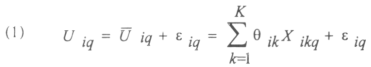As it has been mentioned above, the individual will choose the alternative which maximizes his utility, in other words, the reason why the individual q chooses alternative Ai, is defined as: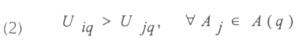Where A(q) symbolizes the group of available alternatives for the individual q. This is like saying that: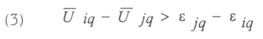As the values of (εiq - εjq) are unknown and stochastic, it is not possible to determine with certainty whether it would result in the inequation (3). Therefore, probabilities should be allocated to the choice of each of the alternatives. Thus, the probability that the individual q chooses the alternative Ai, will be: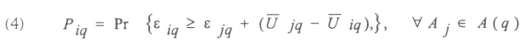In order to estimate such probability, we know that the random variables ε have a specific distribution and based on the assumptions related to it, different models can be generated.

If the non-random term of the equation (1) is: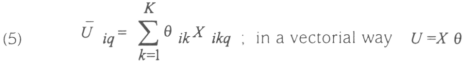Where θik symbolizes the parameters to estimate, and reflects the (non-stochastic) marginal utility of each of the attributes. It should be noticed that the parameters differ in alternative and in attribute, but not in the individual. Although the specified utility function is linear, the model is not; however, it is peculiar since the explanatory variables affect the dependent variable through a linear index (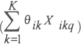), which is then transformed by a distribution function in such a way that the values are consistent with those of a probability.

In case the individual is faced with a choice between two alternatives, the model to be estimated is defined as follows: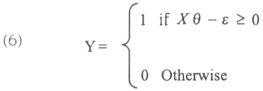Where 0 and 1 symbolizes both alternatives available to the individual, we, therefore, would need to estimate the individual's expected probability of choice on the basis of the attributes corresponding to the choices and the individuals reflected in X.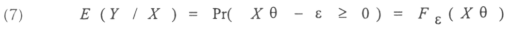Where Fε is the cumulative distribution function.

We will obtain different estimations in accordance with the hypothesis on the distribution of the cumulative distribution function.

2.2. Parametric Estimation of Discrete Choice Models through Logit Models.

The estimation of this type of models, on the assumption that the distribution function is logistic, results in Logit models, which are widely used. On this assumption: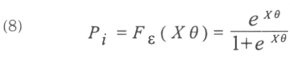The estimation of the parameters θik is performed through the Maximum Likelihood Method. Such method proposes that, although there may be a sample from different populations, there is one population with better probabilities for this to occur. So, the calculated estimators for maximum likelihood consist in a group of parameters, which would generate the observed sample more frequently. McFadden (1975) has demonstrated that the likelihood function of this type of models is well behaved and it has an only maximum if the utility is linear in the parameters similar to the ones mentioned in this paper.

2.3. Identifying interest parameters

The parameters of a model can be identified when, for a given group of observations, the estimators of the said parameters have only one value or, in other words, from a specific sample, there is only one estimator for a given parameter.

In this case, we are interested in estimating the parameters θ . If the probability of choosing the alternative i results form the equation (8), then: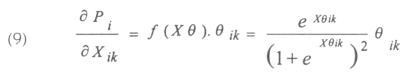Since f(Xθ) is a density function and as such it is always greater than zero, it is possible to determine if the parameters to be estimated will be positive but not their absolute value, because the change in the probability of choice before marginal changes in the attributes X does not have an only answer: it depends on the value of those attributes.

3. Semi-parametric Estimation of Discrete Choice Models

As in the case above, we assume that the cumulative distribution function is logistic, which sometimes may be very restrictive. Accordingly, in this section we will develop an estimation method that does not require any assumption regarding the form of the cumulative distribution function. In order to carry it out, we will construct a simple index model. This model, just as mentioned in the case above, will not allow us to determine the absolute value of the parameters θ , but its average derivative.

It is worth noticing that the estimation proposed in this section is not completely non-parametric, because, even if we leave the distributional assumption aside, we will still maintain the assumption that the probability of choosing any of the alternatives is affected by the vector of attributes X in a linear way3.

For the estimation of the coefficients of the index model we will follow the density weighted average derivatives method presented in Powell et al. (1989) that is a special case of the average derivatives method4. Considering that the problem is estimating the following: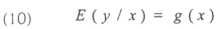Where x is distributed with density f(x), the density weighted average derivative vector is defined as: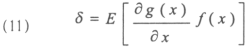Where we are weighting the derivative by the density as if we wanted to estimate the derivative exclusively. If we apply the definition of expectation: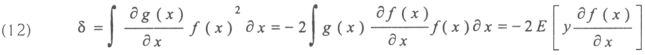Where Y reflects the individual's choice, then, if we adjust the median closer to the average: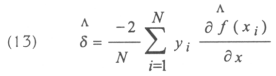Where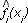is the estimated density of fi(xi), which can get closer in a non-parametric way through Kernel-class estimators, so that: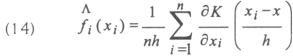The Kernel estimator is a sum of bumps in the observations and the function K determines the shape the bumps will take. Whereas, h stands for the bandwidth size, which is also called smoothing parameter.

The Kernel estimator may take different shapes, though. As it is a weighting function it must be positive and symmetric around zero, so that the point below the median have the same weighting as those that are at the same distance but above the median.

In this paper, we will use a Gaussian Kernel estimator, which is a symmetric density function. In estimating density, this type of Kernel will assign low weighting to the observations more than 3h away from the median. We will also use a Gaussian Kernel estimator that is a symmetric density function. In estimating density, this type of Kernel will assign low weighting to the observations more than 3h away from the median.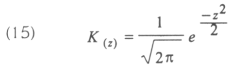Although choosing the Kernel estimator will have influence over the shape of the estimated density, particularly when there are few points and the band is wide, the literature suggests that the choice is not crucial. What is more important is choosing the bandwidth size, since its control is a trade off between bias and variance, while the bias grows, the variance decreases with h.

If h is too big, the estimator are smoothed and turn out to be biased; whereas if h is small, the estimators turn out to be smoothed and their variance is too big5 . Additionally, the values close to the median are better weighted as a result of choosing a small h.

One of the possibilities of choosing the value of h is using an optimal window or, in other words, to minimize the mean square error (defined as the expectation of the integral of the square error over all the density). This was calculated by Silverman (1986) and depends on the actual density and on the Kernel. If we assume that both of them are normal, the optimal window will be: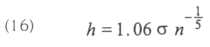3.1 Identifying interest parameters

The value of the density weighted average derivative of equation (10), that is the true equation that we want to estimate, results from the equation (11). Using the definition of expectation and operating mathematically we conclude that: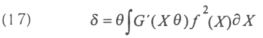Where the integral represented to the right of the equation (17) results in a constant value in a way that: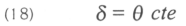Consequently, it is not possible to identify the parameters 9, but the average derivative, since, when calculating the ratio of the estimated values of δ in a parametric way, we obtain the value of the ratio of the parameters θ we want to estimate as the constant term of the equation (18) is cancelled out.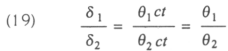4. Measuring Willingness-To-Pay for Transportation Improvements in the City of Buenos Aires

Discrete Choice Models are based on the principle stating that the individual's choice between different alternatives will depend on which one will maximize his utility earnings. Regarding transportation, the alternatives the individual faces are connected to the travel mode he may use. Thus, an individual will rationally choose travelling by the travel mode that maximize his utility.

Therefore, the model to be estimated is intended to explain the modal choice, which means the type of transport chosen by the individual according to the different relevant variables. The model specifications to be used will be the following6:Where, Costingik is the cost of the corresponding mode divided by the individual income (hereinafter denominated "cost"), Timeik represents travel time, Piq is the probability that the individual q chooses one travel mode rather than another i and, finally, θik symbolizes the coefficients of the model to be estimated.

Since the coefficients of the model represent the basis of the non-random term of the utility function given by the equation (1), the marginal rate of substitution (MRS) can result from the ratio of travel time and cost for different alternatives; i.e., the ratio between θi3 and θi2 (which is the average derivative) multiplied by the individual's income would determine the additional cost the individual would be willing to pay for one minute less of travel time, so as the probability of choosing option i is constant. Or, likewise, it would determine his willingness-to-pay for one minute less of travel time so as the non-random term of this utility does not change.

Travel time and cost (as a percentage of the income) are used as explanatory variables of the model. The second variable has been chosen, as opposed to absolute cost value, because it shows, in relative terms, the individual's travel expenditure.

In this case, the options faced by the individual are two: travelling by bus or by subway7. The basic information to be used has been provided by the "Encuesta Domiciliaria de Origen-Destino de Viajes para la Region Metropolitana de Buenos Aires" ["Door to Door O-D Survey of Travel for the Buenos Aires Metropolitan Area"] carried out in 1992. Such information has been updated to the year 2000 considering the re-counting of departure and destination totals, and the distribution of travel has been made by means of the Fratar-Furness mechanism, which led to the construction of a new origin/destination matrix for the year 2000 that includes only the trips made from 8:30 am to 9:30 am. (called "morning rush hour"). So, the model tries to explain the process of modal choice from the pattern of mobility of a representative day in the year 2000 during the morning rush hour.

The different travel fares have been collected by the Comisión Nacional de Regulación del Transporte Automotor [National Commission for the Regulation of Motor Vehicles Transport], whereas the travel times have been obtained from the information provides by the transport companies. It is worth noticing that the individuals have been classified according to the nine categories of income included in the above-mentioned survey8.

4.2. Logit Estimation

Firstly, the model was estimated using a Logit-type parametric specification (Table 1). The estimated parameters θik are shown in the first column, after that, the standard errors of the estimation of each coefficient are detailed, the third column contains the statistical values of individual significance of coefficients and, finally, the fourth column shows the limits of the confidence interval (with a 95% of confidence), including the actual value of the estimated parameter.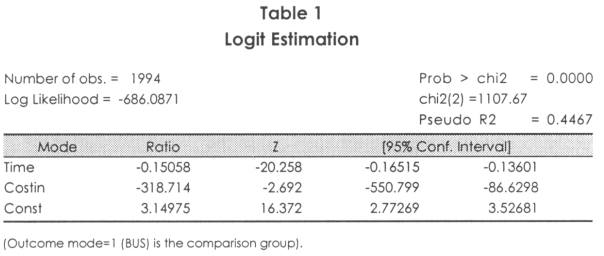The variables are statistically relevant separately and jointly for confidence levels of 99 percent. The advantages of the adjustment can also be studied from the Pseudo statistic R2 and the joint significance test of the coefficients, the specifications corresponding to the former is: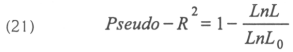Where Ln L is the logarithm of the likelihood function of the original model, i.e., including al the explanatory variables, and Ln L0 is the logarithm of the likelihood function of the restrictive model estimated only with the constant term. Such estimator results in a value of 0.4467, which is acceptable.

Whereas the joint significance test of the coefficients contrasts the null hypothesis θik = 0, against the alternative that a θik 0 exists. The result obtained was chi2 = 1107.67 that, given the distribution chi2, cancels out the null hypothesis of the joint irrelevance of the indicators with confidence levels above 99 percent.

It arises from the estimations that, before increments in subway travel time, there is a reduction in the probability that the individuals choose travelling by subway, and an increase in the probability that they choose travelling by bus, the same happens with the costs as a percentage of the income. The result is utterly intuitive, since the main advantage of travelling by subway (at least in the City of Buenos Aires) is its swiftness and its lower cost, but during the rush hour is rather uncomfortable, which means that before changes in time and costs, the passengers would choose a more comfortable transport mode, such as the bus.

As pointed out, the coefficients of the model are the basis of the non-random term of the utility function given by the equation (1), so that, when calculating the average derivative between θi3 and θi2 from the equation (20) and multiplying it by each individual's level of income you can obtain the MRS between cost and time. Given that 9 categories of income were used, the same number of MRS were obtained for the respective categories. The results are shown in Table 2 and represent the amount of money individuals would be willing to pay for one minute less of subway travel time while the probability of substituting the subway with the bus is constant.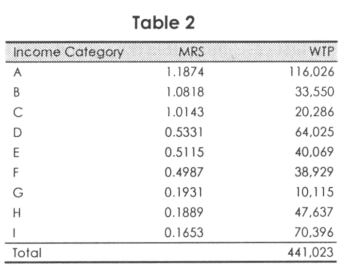On the other hand, you can also estimate the total daily amount of money that all the individuals who travel by subway would be willing to pay in order to reduce their travel time one minute. Consequently, the second column of Table 2 shows the daily willingness-to-pay for one minute less of travel time (WTP), which arises from multiplying the total of daily trips by subway for each income level by the amount of money each individual would be willing to pay.

From Table 2, it can be inferred that the individuals with a higher income -included in category A- would be willing to pay US\$ 1.18 (which, at first, is a really high fare) per minute of reduction in subway travel time, so that the probability of substituting one transport mode with another is constant. As individuals with a lower income are considered, the amounts representing willingness-to-pay for reduced travel time decrease progressively.

A daily amount of US\$ 441,023 results from the aggregation of all the individuals, which represents the total amount of money the individuals would pay per day in order to reduce the travel time one minute, keeping a constant probability of substituting the subway with the bus.

If we consider 24 days per month -since during weekends and bank holidays the traffic is lower- and 12 months per year, the additional money that the total number of passengers who travel by subway would be willing to pay in a year for a reduction of one minute in the travel time, would be US\$ 127.01 million. This shows that the individuals would be willing to finance (at least in theory), through an increase in the travel fare, an annual investment of US\$ 127 million in order to reduce the travel time one minute.

4.3. Non-parametric Estimation of the Modal Choice

For the non-parametric estimation of the average derivative, a Gaussian Kernel estimator was used, and, as explained before, it is important to select the bandwidth size. In this case, as we had more than one explanatory variable, we should choose a bandwidth size for each of them, which (at first) is impossible for estimation purposes, so three different estimations were made considering different values for the bandwidth sizes according to Silverman's suggestion (in 1986), as previously mentioned. The bandwidth sizes were calculated using equation (16) and, considering only the deviation of the variable travel time in the first case, the cost in the second case and, finally, the average between both of them in the third case, the estimations resulted in the following: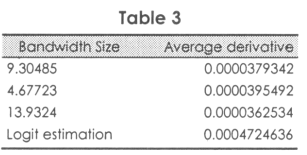It can be noticed that there is no substantial difference among the values for the different bandwidth sizes, but the values do differ with respect to the parametric estimated values.

If the same operation as in the case above is made, we obtain the marginal rates of substitution between cost and travel time multiplying the average derivative by each individual's income. To illustrate it, the first estimation of the average derivative will be used although, given the different magnitudes we are working with, the results would not be significantly different if the other two alternative estimations were used.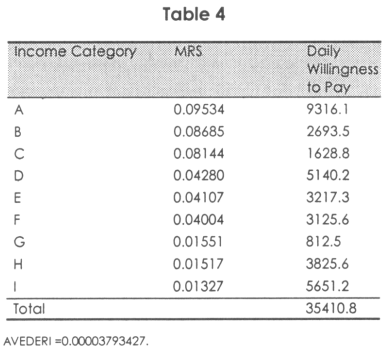It can be observed that the individuals with a higher income would be willing to pay US\$ 0.0953 per minute reduced from travel time, so that the probability of substitution among travel mode is constant. These values are more reasonable than the ones obtained through parametric estimations and reflect international standard values9.

When individuals with a lower income are considered, the amounts representing willingness-to-pay for reduced travel time decrease progressively.

As it happened with the previous estimation, you can also estimate the total daily amount of money that all the individuals who travel by subway would be willing to pay in order to reduce their travel time a minute. The result of the estimation is US\$ 35,410.

Following the previous case, if we consider 24 days per month and 12 months per year, the additional money that the total number of passengers who travel by subway would be willing to pay in a year for a reduction of one minute in the travel time, equals to US\$ 10.2 million. This shows that the individuals would be willing to finance (at least in theory), through an increase in the travel fare, an annual investment of only US\$ 10.2 million in order to reduce the travel time one minute. These values are utterly different from the ones obtained in the parametric estimation.

5. Conclusions

Two alternative estimations for a discrete choice model regarding the choice of the travel mode in the City of Buenos Aires have been carried out, using parametric and non-parametric techniques which resulted in significant differences.

It should be emphasized that the measures used for adjustment in Logit models had positive results, which would imply that, at first, the specification of the models was correct. However, given that one of the disadvantages of this type of models is that their distributional assumptions are so restrictive that, whenever they are inaccurate, they can lead to biased estimations, a non-parametric estimation was carried out using a simple index model whose results were substantially different.

In order to illustrate the significance of the potential errors incurred when estimating with too restrictive assumptions, willingness-to-pay for improved subway travel times was estimated. Through this estimation it was concluded that, as regards the parametric case, the total of passengers would be willing to pay US\$ 127 million for one minute less of travel time, and according to the semi-parametric estimation, willingness-to-pay was only US\$ 10.2 million.

Consequently, if a Logit model is used, the advised policy would be to increase the subway fare up to a US\$ 127 million-investment were financed in order to reduce the travel time one minute; whereas the non-parametric estimation would suggest that passengers are willing to finance only US\$ 10.2 million by means of increases in the fares.

Although these results are exclusively shown for illustrative purposes since segregated and updated information regarding the topic is not available, the significance of the differences found emphasizes the need to examine this type of problems.

Notes

* Inter.-American Development Bank

The author would like to thank an anonymous referee for many useful comments and suggestions. His thanks go also to Rosa Matzkin, Jonn Tindall, Mariana Conte Grand, Luis Rizzi and Alfredo Canavese for constructive comments on an earlier version of the paper. Remaining errors are the author's sole responsibility.

** La traducción del resumen del inglés al español es responsabilidad del comité de editores de la Revista Latinoamericana de Desarrollo Económico.

1      For a review of the literature on willingness-to-pay see Hanemann and Kanninen (2002). Bicknell (2001) or Mc Fadden (1997) for transportation applications.

2     In order to analyze hypothetical markets, fhe stated preference theory is generally used, since the hypothetica feature of the market, individuals can not possible reveal their preferences, as a result, there are techniques to examine the likely individuals behavior on such market.

3      In 1992, Matzkin introduced a completely non-parametric way of estimating for this type of models.

4      For an introduction to the average derivative method see Hardle and Stocker (1989).

5      Additionally, the values close to the median are better weighted as a result of choosing a small h.

6      The literature regarding transportation also points out that there are other relevant variables can explain the process of the modal choice. These variables include the waiting time, the distance between the individual's home and the station, or bus stop, whether the individual have a car or not, etc. For further details, see Ben-Akiva y Lerman (1985). Fortunately, this type of information is not available for the case examined in this paper.

7      In order to take the sample, it was considered whether the individual had access to both means of transport, so that one could be replaced by the other.

8      In Garcia (2002), you can find a detailed description of the database used and the incomes of each category .

9      Department of Transport (1985) "Traffic Appraisal Manual".

REFERENCES

Amemiya, T. 1995. "Advanced Econometrics". Cambridge Massachussecs: Harvard University Press.

Ben-Akiva, M. and S. Lerman. 1985. "Discrete Choice Analysis: Theory and Application to Travel Demand". Cambridge, Massachusetts: MIT Press.

Bicknell, K. 2001. "Willingness-To-Pay for the Benefits of Environmental Improvement: A Literature Review and Some Recommendations". Environment Canterbury Report N° U01/89

García, Pablo M. 2002. "Una aproximación microeconométrica a los determinantes de la elección del modo de transporte". Annals of the XXXVII Annual Meeting of the Argentine Association of Political Economy.

Hanemann, M and B . Kanninen. 2002. "The Statistical Analysis of Discrete-Response CV Data". In: Ian J. Bateman and Ken Willis (eds.).Valuing Environmental Preferences: Theory and Practice of the Contingent Valuation Method in the US, EU. and Developing Countries. Oxford University Press.

Hardle, W and T . Stoker. 1989. "Investigating Smooth Multiple Regression by the Method of Average Derivatives". Journal of the American Statistical Association. Vol 84. N°. 408. Matzkin, R. 1992. "Non-parametric and Distribution-Free Estimation of the Binary Threshold Crossing and the Binary Choice Models". Econometrica. Vol 60, No. 2.

McFadden, Daniel. 1975. "The Measurement of Urban Travel Demand". University of California, Berkeley.

                  1997. "Measuring Willingness-To-Pay for Transportation Improvements". University of California, Berkeley.

Ortúzar J. D. and L. G. Willumsen. 1994. Modelling Transport. UK: John Wiley &. Sons. Second Edition.

Ortúzar J. D. 2000. "Modelos econométricos de elección discreta". Pontificia Universidad Católica de Chile.

Pagan, A. and A. Ullah. 1999. "Nonparametric Econometrics". Cambridge University Press.

Powel, J., J. Stock and T. Stoker. 1 989. "Semiparametric Estimation of Index Coefficients". Econometrica Vol. 57, N° 6.

Silverman, B. 1986. Density Estimation for Statistics and Data Analysis. London and New York: Chapman and Hall.

Willumsen, L. 1994. "Uso de preferencias declaradas para estimar el valor de la calidad de servicio" [Using Revealed Preferences to Estimate the Value of Service Quality] VII Latin American Congress of Public and Urban Transportation. Buenos Aires.Todo el contenido de esta revista, excepto dónde está identificado, está bajo una Licencia Creative Commons